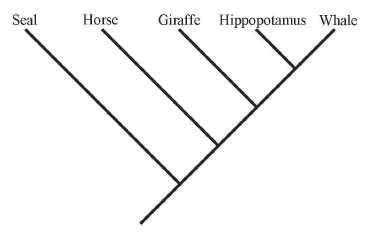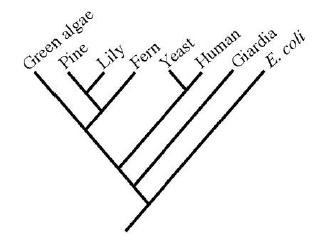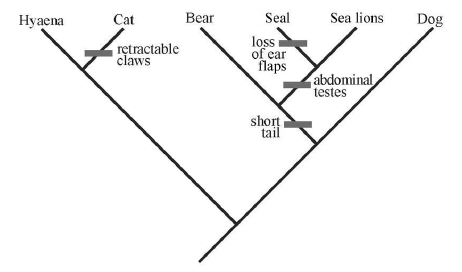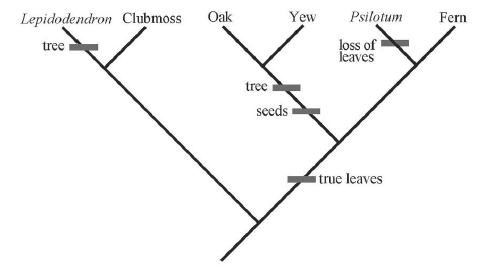# 10.6: Lab 9 report

$$\newcommand{\vecs}{\overset { \rightharpoonup} {\mathbf{#1}} }$$ $$\newcommand{\vecd}{\overset{-\!-\!\rightharpoonup}{\vphantom{a}\smash {#1}}}$$$$\newcommand{\id}{\mathrm{id}}$$ $$\newcommand{\Span}{\mathrm{span}}$$ $$\newcommand{\kernel}{\mathrm{null}\,}$$ $$\newcommand{\range}{\mathrm{range}\,}$$ $$\newcommand{\RealPart}{\mathrm{Re}}$$ $$\newcommand{\ImaginaryPart}{\mathrm{Im}}$$ $$\newcommand{\Argument}{\mathrm{Arg}}$$ $$\newcommand{\norm}{\| #1 \|}$$ $$\newcommand{\inner}{\langle #1, #2 \rangle}$$ $$\newcommand{\Span}{\mathrm{span}}$$ $$\newcommand{\id}{\mathrm{id}}$$ $$\newcommand{\Span}{\mathrm{span}}$$ $$\newcommand{\kernel}{\mathrm{null}\,}$$ $$\newcommand{\range}{\mathrm{range}\,}$$ $$\newcommand{\RealPart}{\mathrm{Re}}$$ $$\newcommand{\ImaginaryPart}{\mathrm{Im}}$$ $$\newcommand{\Argument}{\mathrm{Arg}}$$ $$\newcommand{\norm}{\| #1 \|}$$ $$\newcommand{\inner}{\langle #1, #2 \rangle}$$ $$\newcommand{\Span}{\mathrm{span}}$$$$\newcommand{\AA}{\unicode[.8,0]{x212B}}$$

1. Write your dinosaur character list and draw the character table below. How did you determine which character state is plesiomorphic and which is apomorphic? Explain. (5 pts).

Then, draw two of your dinosaur phylogeny trees (if there is no space, use reverse side or add one page). Do not forget to label all apomorphies and reversals (if any). Note the length of trees. Circle the most parsimonious tree. Why is this tree most parsimonious? Explain. (5 pts).

2. On next pages, answer basic three thinking assignment (5 pts, 1/2 point per answer).

Basic Tree Thinking Assessment

David A. Baum, Stacey DeWitt Smith, Samuel S. Donovan

This quiz includes a number of multiple-choice questions you can use to test yourself on your ability to accurately interpret evolutionary trees. Insofar as real biological examples have been used they are accurate based on current knowledge.Figure $$\PageIndex{1}$$
1. By reference to the tree above, which of the following is an accurate statement of relationships?
1. A green alga is more closely related to a red alga than to a moss
2. A green alga is more closely related to a moss than to a red alga
3. A green alga is equally related to a red alga and a moss
4. A green alga is related to a red alga, but is not related to a mossFigure $$\PageIndex{2}$$
1. By reference to the tree above, which of the following is an accurate statement of relationships?
1. A crocodile is more closely related to a lizard than to a bird
2. A crocodile is more closely related to a bird than to a lizard
3. A crocodile is equally related to a lizard and a bird
4. A crocodile is related to a lizard, but is not related to a birdFigure $$\PageIndex{3}$$
1. By reference to the tree above, which of the following is an accurate statement of relationships?
1. A seal is more closely related to a horse than to a whale
2. A seal is more closely related to a whale than to a horse
3. A seal is equally related to a horse and a whale
4. A seal is related to a whale, but is not related to a horseFigure $$\PageIndex{4}$$
1. Which of the five marks in the tree above corresponds to the most recent common ancestor of a mushroom and a sponge?Figure $$\PageIndex{5}$$
1. If you were to add a trout to the phylogeny shown above, where would its lineage attach to the rest of the tree?Figure $$\PageIndex{6}$$
1. Which of trees below is false given the larger phylogeny above?Figure $$\PageIndex{7}$$Figure $$\PageIndex{8}$$
1. Which of the four trees above depicts a different pattern of relationships than the others?Figure $$\PageIndex{9}$$
1. Which of the four trees above depicts a different pattern of relationships than the others?Figure $$\PageIndex{10}$$
1. In the above tree, assume that the ancestor had a long tail, ear flaps, external testes, and fixed claws. Based on the tree and assuming that all evolutionary changes in these traits are shown, what traits does a sea lion have?
1. long tail, ear flaps, external testes, and fixed claws
2. short tail, no ear flaps, external testes, and fixed claws
3. short tail, no ear flaps, abdominal testes, and fixed claws
4. short tail, ear flaps, abdominal testes, and fixed claws
5. long tail, ear flaps, abdominal testes, and retractable clawsFigure $$\PageIndex{11}$$
1. In the above tree, assume that the ancestor was a herb (not a tree) without leaves or seeds. Based on the tree and assuming that all evolutionary changes in these traits are shown, which of the tips has a tree habit and lacks true leaves?
1. Lepidodendron
2. Clubmoss
3. Oak
4. Psilotum
5. Fern

This page titled 10.6: Lab 9 report is shared under a Public Domain license and was authored, remixed, and/or curated by Alexey Shipunov.